# Fractals

## Mandelbrot set

The Mandelbrot set is the set complex numbers for which the function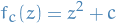does not diverge when iterated from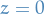, i.e. remains bounded in absolute value.

Formally, the Mandelbrot set is the set of values of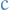in the complex plane for which the orbit of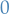under iteration of the quadratic map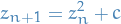remains bounded.

The Mandelbrot set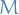is defined by a family of complex quadratic polynomials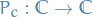given by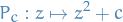whereis a complex parameter. For each, one considers the behavior of the sequence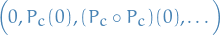obtained by iteration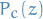starting at critical point. This either escapes to infinity or stays within a disk of finite radius; the Mandelbrot set is then the set of all pointssuch that the sequence stays within a disk of finite radius.

More formally, if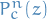denotes the n-th iterate of(i.e.composed with itself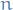times), the Mandelbrot set is the subset of the complex plane given by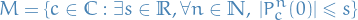As explained below, it is in fact possible to simplify this definition by taking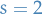.

### Visualizations of Mandelbrot sets

• Color all pointsthat belong to the Mandelbrot setblack and all other points white

### Connection to Julia sets

An equivalent definition of the Mandelbrot set is as the subset of the complex plane consisting of those parametersfor which the Julia set of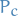is connected.

## Julia set

Let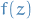be a holomorphic function from the Riemann sphere to itself.

Suchare precisely the complex rational functions, that is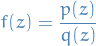where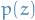and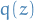are complex polynomials.

Assume that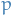and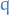are non-constant, and that they have no common roots (i.e.does not divide). Then there is a finite number of open sets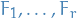, that are left invariant byand are such that:

1.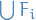is dense in the plane
2.behaves in a regular and equal way on each of the sets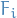The last statement means that the termini of the sequences of iterations generated by the points ofare either

• the same set => finite cycle
•are finite cycles of circular or annular shaped sets centered at the same point

These setsare the Fatou domains of, and their union is the Fatou set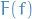of.

Further, each of the Fatou domains contains at least on critical point of, i.e. a (finite) point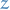such that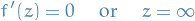if either of the following is the case:

• degree of the numeratoris at least two larger than the degree of the denominator•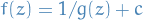for someand a rational function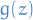satisfying this condition

The complement of the Fatou setis the Julia set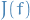of.is a nowhere dense set (it is without interior points) and an uncountable set (of the same cardinality as the real numbers).

Like,is left invariant by, and on this set the iteration is repelling, meaning that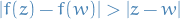for all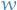in a neighborhood of(within).

This means thatbehaves chaotically on the Julia set.

There are points in the Julia set whose sequence of iterations is finite, there are only a countable number of such points (and thus they only make up a infinitely small part of the Julia set). The sequences generated by points outside this set behave chaotically, a phenomenon called deterministic chaos.

Equivalent definitions of Julia sets are:

•is the smallest closed set containing at least three points which is completely invariant under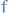(Möbius transformation?)
•is the closure of the set of repelling periodic points
• For all but at most two points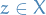, the Julia set is the set of limit points of the full backwards orbit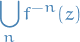i.e. limit points of the union of pre-images of all the iterations.

• Ifis an entire function, thenis the boundary of the set of points which converge to infinity under iteration.
• Ifis a polynomial, thenis the boundary of the filled Julia set: i.e. those points whose orbits under iterations ofremain bounded.

### Julia set of a Mandelbrot set

Mandelbrot sets are given bywhereis a complex parameter.

The Julia set of for this system is the subset of complex plane given by: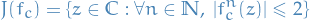where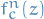is the n-th iterator of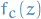, i.e.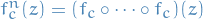, n times.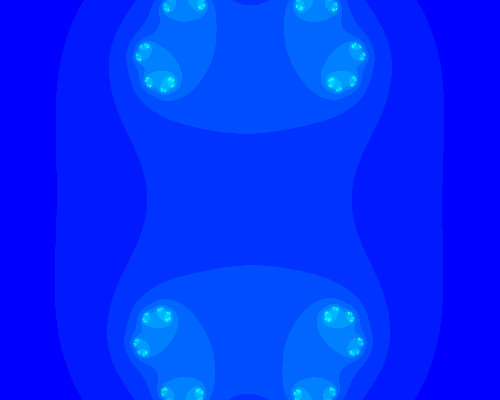Figure 1: Source: https://www.wikiwand.com/en/Julia_set